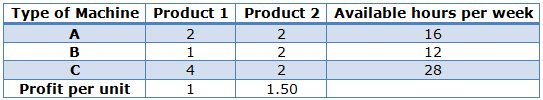# Methods of Solving: Graphical and Simplex

The Graphical Method (graphic solving) is an excellent alternative for the representation and solving of Linear Programming models that have two decision variables.

Exercise #1: A workshop has three (3) types of machines A, B and C; it can manufacture two (2) products 1 and 2, and all products have to go to each machine and each one goes in the same order; First to the machine A, then to B and then to C. The following table shows:

• The hours needed at each machine, per product unit
• The total available hours for each machine, per week
• The profit of each product per unit soldFormulate and solve using the graphical method a Linear Programming model for the previous situation that allows the workshop to obtain maximum gains.

Decision Variables

• Product 1 Units to be produced weekly
• Product 2 Units to be produced weekly

Objective Function

Maximize2

Constraints:

The constraints represent the number of hours available weekly for machines A, B and C, respectively, and also incorporate the non-negativity conditions.

For the graphical solution of this model we will use the Graphic Linear Optimizer (GLP) software. The green colored area corresponds to the set of feasible solutions and the level curve of the objective function that passes by the optimal vertex is shown with a red dotted line.The optimal solution is  and  with an optimal value  that represents the workshop’s profit.

### SIMPLEX METHOD

The Simplex Method or Simplex Algorithm is used for calculating the optimal solution to the linear programming problem. In other words, the simplex algorithm is an iterative procedure carried systematically to determine the optimal solution from the set of feasible solutions.

Firstly, to apply the simplex method, appropriate variables are introduced in the linear programming problem, and the primary or the decision variables are equated to zero. The iterative process begins by assigning values to these defined variables. The value of decision variables is taken as zero since the evaluation in terms of the graphical approach begins with the origin. Therefore, x1 and x2 is equal to zero.

The decision maker will enter appropriate values of the variables in the problem and find out the variable value that contributes maximum to the objective function and removes those values which give undesirable results. Thus, the value of the objective function gets improved through this method. This procedure of substitution of variable value continues until any further improvement in the value of the objective function is possible.

Following two conditions need to be met before applying the simplex method:

1. The right-hand side of each constraint inequality should be non-negative. In case, any linear programming problem has a negative resource value, then it should be converted into positive value by multiplying both the sides of constraint inequality by “-1”.
2. The decision variables in the linear programming problem should be non-negative.

Thus, the simplex algorithm is efficient since it considers few feasible solutions, provided by the corner points, to determine the optimal solution to the linear programming problem.

## One thought on “Methods of Solving: Graphical and Simplex ”

error: Content is protected !!